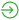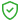Current Status
Not Enrolled
Price
Closed
Get Started
This test is currently closed

## The Numerical Reasoning practice pack includes:

• Basic Mathematics
• Word Problems
• Numerical Reasoning
• Numerical Critical Reasoning
• Numerical Series6 months access24/7 Customer Support* 30-Day Money-Back Guarantee

One of the most popular psychometric tests used by companies to find suitable employees is a numerical reasoning test. Psychometric exams are well-researched and reliable exams that psychologists have thoroughly tested to help employers evaluate your potential job performance.

Numerical reasoning tests are often found in job applications involving numerical data, such as accounting, data analysis, finances, and insurance, though they are becoming more common in marketing and human relations positions.

Unlike math tests, psychometric tests don’t ask that you memorize equations to answer the questions. Instead, you must be able to use the numerical information given within the examination to find solutions to problems relevant to the desired job role.

## What is a Numerical Reasoning Test?

Numerical reasoning tests assess how well you apply reasoning to numerical scenarios within the workplace. Your ability to analyze and interpret finances, convert currency, predict number sequences, use basic mathematical arithmetic, and more are examined.

There is no generic numerical reasoning test, so each test differs from the next. The type of test you will encounter largely depends on the company and job role in which you are applying. Roughly speaking, numerical reasoning tests can last anywhere from 12 to 25 minutes and are multiple-choice.

The most common numerical reasoning tests are issued by test providers SHLRevelian, and Talent Q, though there are many more. If possible, ask the employer which test provider is used for your numerical reasoning test so that you can prepare for that exam.

## How Your Numerical Reasoning Test is Scored

You will receive your results immediately after completing the test. Your score is calculated based on how well you perform compared to a sample of previous test-takers. The average percentile is 50. Scoring in a higher percentile means you’ve done better than average within the group, while scoring lower means you’ve scored less than average.

Many companies use the 40th percentile as the lowest possible score to move onto the next round of the application process, although depending on the complexity and difficulty of the job position, this cut-off score might be higher. In order to not be cut off from the next step, aim for a passing score for that particular numerical reasoning test.

## Common Numerical Reasoning Question Types

Familiarizing yourself with the most popular numerical reasoning questions prior to the test day is a sure-fire way to set yourself up for success. Below are 4 common topics that are likely to appear on the test.

### Basic Arithmetic

In the basic arithmetic section, your knowledge of basic numerical computations is assessed. Calculations involving addition, subtraction, division, and multiplication are common in these kinds of questions, as well as calculating percentages, ratios, and proportions.

### Data Interpretation

Data interpretation involves using images and text to analyze data and form inferences based on the information given. The main goal of these questions is to see how well you can use limited information to form conclusions.

• Graphs

Line and bar graphs display information in which you must analyze the relationship between the given variables.

• Tables

Rows and columns display information in which you must use to answer relevant questions.

• Written text

Using written text, you will need to extract data to answer related questions.

### Number Series

In numerical series questions, your aptitude in numerical logic is examined. Instead of looking at how well you can calculate basic mathematics, this section looks at whether or not you can use logic to solve for missing numbers.

You will be presented with a number sequence in which one number is missing. Your job is to figure out the relationship between the numbers and apply that operational rule to find the missing number. These types of questions get increasingly more difficult as you take the test.

### Currency and Unit Conversion

This section assesses your ability to convert currency or units into different values. These questions often are displayed in tables or graphs.

How to Pass a Numerical Reasoning Test

To pass your numerical reasoning test, there are a few ways that you can prepare for success:

• Take numerical reasoning practice tests to get familiar with your strengths and weaknesses.
• Study the areas that you struggle with the most.
• Time your practice questions to get used to working under pressure.
• Find out which test provider the employer is using to prepare for the correct test.
• Arrive early for your test so that you can take a few moments to breathe and focus.

Ultimately, the best way to score high on your numerical reasoning test is to take a practice exam that covers all that you might see on your test. Our numerical reasoning practice test has everything you need to succeed on your exams. Reach out to us today to get started.

Start practicing today to improve your score on the real test.

Categories: